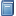Buchempfehlung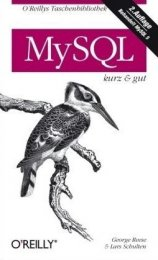MySQL kurz & gut
Das preiswerte Taschen- buch stellt MySQL-rele- vante Inhalte systematisch und knapp dar, sodass es sich optimal zum Nach- schlagen beim Pro- grammieren eignet. [Mehr Infos...]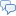FreeBASIC-Chat
Es sind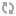Benutzer im FreeBASIC-Chat online.
(Stand:  )

# fb:porticula NoPaste

## TSNEplay_V3.bi

 Uploader: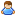ThePuppetMaster Datum/Zeit: 06.01.2013 14:18:58
Hinweis: Dieser Quelltext ist Bestandteil des Projekts TSNEPlay, zu dem es auf FreeBASIC-Portal.de eine Projektseite gibt.

```'############################################################################################################## '############################################################################################################## ' TSNEplay_V3 - TCP Socket Networking [Eventing] Play (Gaming Extension) Version: 1.0 for TSNE_V3 '############################################################################################################## '############################################################################################################## ' 2009 By.: /_\ DeltaLab's Germany - Experimental Computing ' Autor: Martin Wiemann '############################################################################################################## #IFNDEF _TSNEplay_     #DEFINE _TSNEplay_ '>... '############################################################################################################## #define TSNE_DEF_REUSER #Include once "TSNE_V3.bi" '############################################################################################################## Enum TSNEPlay_GURUCode     TSNEPlay_NoError                            = 1     TSNEPlay_Unknown                            = 0     TSNEPlay_NotReadyForNewConnection           = -1000     TSNEPlay_PlayerIDNotFound                   = -1001     TSNEPlay_MessageToLong                      = -1002 End Enum '############################################################################################################## Enum TSNEPlay_State_Enum     TSNEPlay_State_Unknown                      = 0     TSNEPlay_State_Disconnected                 = 1     TSNEPlay_State_Connecting                   = 2     TSNEPlay_State_Connected                    = 3     TSNEPlay_State_Login                        = 4     TSNEPlay_State_Ready                        = 5 End Enum '-------------------------------------------------------------------------------------------------------------- Enum TSNEPlay_MessageType_Enum     TSNEPlay_MSGType_Regular                    = 0     TSNEPlay_MSGType_Private                    = 1     TSNEPlay_MSGType_Notice                     = 2     TSNEPlay_MSGType_Hightlighted               = 3 End Enum '############################################################################################################## Enum TSNEPlay_INT_CMDType_Enum     TSNEPlay_INT_MSGT_Unknown                   = 0     TSNEPlay_INT_MSGT_NeedPassword              = 1     TSNEPlay_INT_MSGT_Password                  = 2     TSNEPlay_INT_MSGT_PasswordWrong             = 3     TSNEPlay_INT_MSGT_GetInfo                   = 4     TSNEPlay_INT_MSGT_PutInfo                   = 5     TSNEPlay_INT_MSGT_Ready                     = 10     TSNEPlay_INT_MSGT_StreamError               = 999999     TSNEPlay_INT_MSGT_Ping                      = 1000     TSNEPlay_INT_MSGT_Pong                      = 1001     TSNEPlay_INT_MSGT_MSG                       = 1002     TSNEPlay_INT_MSGT_Move                      = 1003     TSNEPlay_INT_MSGT_Dat                       = 1004     TSNEPlay_INT_MSGT_Con                       = 1005     TSNEPlay_INT_MSGT_Dis                       = 1006     TSNEPlay_INT_MSGT_Full                      = 1007 End Enum '############################################################################################################## Type TSNEPlay_INT_CommandQue_Type     V_Next                                          as TSNEPlay_INT_CommandQue_Type Ptr     V_Prev                                          as TSNEPlay_INT_CommandQue_Type Ptr     V_RAW                                           as String     V_CMDType                                       as TSNEPlay_INT_CMDType_Enum     V_Data                                          as String End Type '############################################################################################################## Type TSNEPlay_INT_Client_Type     V_Next                                          as TSNEPlay_INT_Client_Type Ptr     V_Prev                                          as TSNEPlay_INT_Client_Type Ptr     V_TSNEID                                        as UInteger     V_IPA                                           as String     V_Data                                          as String     V_State                                         as TSNEPlay_State_Enum     V_CMDQueF                                       as TSNEPlay_INT_CommandQue_Type Ptr     V_CMDQueL                                       as TSNEPlay_INT_CommandQue_Type Ptr     V_CMDQueC                                       as UShort     V_PlayerID                                      as UInteger     V_Nickname                                      as String     T_LastPing                                      as Double     T_PingT                                         as Double End Type '-------------------------------------------------------------------------------------------------------------- Dim Shared TSNEPlay_INT_ClientF                     as TSNEPlay_INT_Client_Type Ptr Dim Shared TSNEPlay_INT_ClientL                     as TSNEPlay_INT_Client_Type Ptr Dim Shared TSNEPlay_INT_ClientC                     as UInteger Dim Shared TSNEPlay_INT_PlayerIDC                   as UInteger '############################################################################################################## Dim Shared TSNEPlay_INT_Mutex                       as Any Ptr '-------------------------------------------------------------------------------------------------------------- Dim Shared TSNEPlay_INT_State                       as TSNEPlay_State_Enum Dim Shared TSNEPlay_INT_ClientMode                  as UByte Dim Shared TSNEPlay_INT_ServerPassword              as String Dim Shared TSNEPlay_INT_Nickname                    as String '-------------------------------------------------------------------------------------------------------------- Dim Shared TSNEPlay_INT_Server_TSNEID               as UInteger Dim Shared TSNEPlay_INT_Server_PlayerID             as UInteger Dim Shared TSNEPlay_INT_Server_MaxPlayer            as UShort '-------------------------------------------------------------------------------------------------------------- Dim Shared TSNEPlay_INT_Client_TSNEID               as UInteger Dim Shared TSNEPlay_INT_Client_Data                 as String Dim Shared TSNEPlay_INT_Client_PlayerID             as UInteger '-------------------------------------------------------------------------------------------------------------- Dim Shared TSNEPlay_INT_Event_ConnectionState       as Sub (ByVal V_FromPlayerID as UInteger, ByVal V_State as TSNEPlay_State_Enum) Dim Shared TSNEPlay_INT_Event_Player_Connected      as Sub (ByVal V_PlayerID as UInteger, V_IPA as String, V_Nickname as String) Dim Shared TSNEPlay_INT_Event_Player_Disconnected   as Sub (ByVal V_PlayerID as UInteger) Dim Shared TSNEPlay_INT_Event_Message               as Sub (ByVal V_FromPlayerID as UInteger, ByVal V_ToPlayerID as UInteger, ByVal V_Message as String, ByVal V_MessageType as TSNEPlay_MessageType_Enum) Dim Shared TSNEPlay_INT_Event_Move                  as Sub (ByVal V_FromPlayerID as UInteger, ByVal V_ToPlayerID as UInteger, ByVal V_NewPositionX as Double, ByVal V_NewPositionY as Double, ByVal V_NewPositionZ as Double, ByVal V_SubData as UInteger) Dim Shared TSNEPlay_INT_Event_Data                  as Sub (ByVal V_FromPlayerID as UInteger, ByVal V_ToPlayerID as UInteger, ByRef V_Data as String) '############################################################################################################## Sub TSNEPlay_INT_Construct() Constructor TSNEPlay_INT_Mutex = MutexCreate() End Sub '-------------------------------------------------------------------------------------------------------------- Sub TSNEPlay_INT_Destruct() Destructor MutexDestroy(TSNEPlay_INT_Mutex) TSNEPlay_INT_Mutex = 0 End Sub '############################################################################################################## Function TSNEPlay_INT_ClientGetPID(V_PlayerID as UInteger) as TSNEPlay_INT_Client_Type Ptr Dim TPtr as TSNEPlay_INT_Client_Type Ptr = TSNEPlay_INT_ClientF Do Until TPtr = 0     If TPtr->V_PlayerID = V_PlayerID Then Return TPtr     TPtr = TPtr->V_Next Loop Return 0 End Function '-------------------------------------------------------------------------------------------------------------- Function TSNEPlay_INT_ClientGetTSNEID(V_TSNEID as UInteger) as TSNEPlay_INT_Client_Type Ptr Dim TPtr as TSNEPlay_INT_Client_Type Ptr = TSNEPlay_INT_ClientF Do Until TPtr = 0     If TPtr->V_TSNEID = V_TSNEID Then Return TPtr     TPtr = TPtr->V_Next Loop Return 0 End Function '-------------------------------------------------------------------------------------------------------------- Function TSNEPlay_INT_ClientAdd() as TSNEPlay_INT_Client_Type Ptr Dim TPtr as TSNEPlay_INT_Client_Type Ptr TSNEPlay_INT_PlayerIDC += 1 Do Until TSNEPlay_INT_ClientGetPID(TSNEPlay_INT_PlayerIDC) = 0     TSNEPlay_INT_PlayerIDC += 1     If TSNEPlay_INT_PlayerIDC = 0 Then TSNEPlay_INT_PlayerIDC = 1 Loop If TSNEPlay_INT_ClientL <> 0 Then     TSNEPlay_INT_ClientL->V_Next = CAllocate(SizeOf(TSNEPlay_INT_Client_Type))     TSNEPlay_INT_ClientL->V_Next->V_Prev = TSNEPlay_INT_ClientL     TSNEPlay_INT_ClientL = TSNEPlay_INT_ClientL->V_Next Else     TSNEPlay_INT_ClientL = CAllocate(SizeOf(TSNEPlay_INT_Client_Type))     TSNEPlay_INT_ClientF = TSNEPlay_INT_ClientL End If With *TSNEPlay_INT_ClientL     .V_PlayerID = TSNEPlay_INT_PlayerIDC End With TSNEPlay_INT_ClientC += 1 Return TSNEPlay_INT_ClientL End Function '-------------------------------------------------------------------------------------------------------------- Sub TSNEPlay_INT_ClientDel(V_Client as TSNEPlay_INT_Client_Type Ptr) If V_Client->V_Next <> 0 Then V_Client->V_Next->V_Prev = V_Client->V_Prev If V_Client->V_Prev <> 0 Then V_Client->V_Prev->V_Next = V_Client->V_Next If TSNEPlay_INT_ClientF = V_Client Then TSNEPlay_INT_ClientF = V_Client->V_Next If TSNEPlay_INT_ClientL = V_Client Then TSNEPlay_INT_ClientL = V_Client->V_Prev With *V_Client     Do Until .V_CMDQueF = 0         .V_CMDQueL = .V_CMDQueF->V_Next         DeAllocate(.V_CMDQueF)         .V_CMDQueF = .V_CMDQueL     Loop End With DeAllocate(V_Client) If TSNEPlay_INT_ClientC > 0 Then TSNEPlay_INT_ClientC -= 1 End Sub '############################################################################################################## Function TSNEPlay_Desc_GetGuruCode(V_GuruCode as TSNEPlay_GURUCode) as String Select Case V_GuruCode     Case TSNEPlay_NoError                           : Return "No error!"     Case TSNEPlay_Unknown                           : Return "Unknown error!"     Case TSNEPlay_NotReadyForNewConnection          : Return "Not Ready! Conection Already Exist! Close all connections!"     Case TSNEPlay_MessageToLong                     : Return "Message text is too long!"     Case Else                                       : Return TSNE_GetGURUCode(V_GuruCode) End Select End Function '-------------------------------------------------------------------------------------------------------------- Function TSNEPlay_Desc_GetStateCode(V_State as TSNEPlay_State_Enum) as String Select Case V_State     Case TSNEPlay_State_Unknown                     : Return "Unknown"     Case TSNEPlay_State_Disconnected                : Return "Disconnected!"     Case TSNEPlay_State_Connecting                  : Return "Connecting..."     Case TSNEPlay_State_Connected                   : Return "Connected!"     Case TSNEPlay_State_Login                       : Return "Login..."     Case TSNEPlay_State_Ready                       : Return "Ready!"     Case Else                                       : Return "[Unknown State-Code]" End Select End Function '############################################################################################################## Function TSNEPlay_INT_CreateStreamCommand(V_Command as TSNEPlay_INT_CMDType_Enum, V_Data as String = "") as String 'Print "OUT_CMD: >"; Str(V_Command); "<___>"; Str(Len(V_Data)) & "<" Dim MX as UInteger = 4 + Len(V_Data) Dim T as String T += Chr((MX shr 24) and 255) & Chr((MX shr 16) and 255) & Chr((MX shr 8) and 255) & Chr(MX and 255) T += Chr((V_Command shr 24) and 255) & Chr((V_Command shr 16) and 255) & Chr((V_Command shr 8) and 255) & Chr(V_Command and 255) Return T & V_Data End Function '-------------------------------------------------------------------------------------------------------------- Sub TSNEPlay_INT_ParseStreamCommand(ByRef V_Data as String, ByRef R_CMDQueF as TSNEPlay_INT_CommandQue_Type Ptr, ByRef R_CMDQueL as TSNEPlay_INT_CommandQue_Type Ptr) Dim MX as UInteger Dim XRAW as String Dim XCMD as TSNEPlay_INT_CMDType_Enum Dim XData as String Do     If Len(V_Data) < 8 Then Exit Sub     MX = (V_Data shl 24) or (V_Data shl 16) or (V_Data shl 8) or V_Data     If (Len(V_Data) - 4) < MX Then Exit Sub     XCMD = (V_Data shl 24) or (V_Data shl 16) or (V_Data shl 8) or V_Data     XRAW = Left(V_Data, MX + 4)     XData = Mid(V_Data, 9, MX - 4)     V_Data = Mid(V_Data, MX + 5)     If R_CMDQueL <> 0 Then         R_CMDQueL->V_Next = CAllocate(SizeOf(TSNEPlay_INT_CommandQue_Type))         R_CMDQueL = R_CMDQueL->V_Next     Else         R_CMDQueL = CAllocate(SizeOf(TSNEPlay_INT_CommandQue_Type))         R_CMDQueF = R_CMDQueL     End If     With *R_CMDQueL         .V_RAW = XRAW         .V_CMDType = XCMD         .V_Data = XData     End With Loop End Sub '-------------------------------------------------------------------------------------------------------------- Function TSNEPlay_INT_SendData(V_ToPlayerID as UInteger, V_Data as String, ByRef R_LocalSend as UByte) as TSNEPlay_GURUCode 'Print "OUT:"; V_ToPlayerID; " "; Len(V_Data); "  ";: For XXX as UInteger = 1 to Len(V_Data): Print V_Data[XXX - 1]; " ";: Next: Print R_LocalSend = 0 If TSNEPlay_INT_ClientMode = 1 Then     TSNE_Data_Send(TSNEPlay_INT_Client_TSNEID, V_Data)     Return TSNEPlay_NoError End If Dim TPtr as TSNEPlay_INT_Client_Type Ptr MutexLock(TSNEPlay_INT_Mutex) If V_ToPlayerID > 0 Then     Dim TSID as UInteger     TPtr = TSNEPlay_INT_ClientGetPID(V_ToPlayerID)     If TPtr = 0 Then MutexUnLock(TSNEPlay_INT_Mutex): Return TSNEPlay_PlayerIDNotFound     TSID = TPtr->V_TSNEID     MutexUnLock(TSNEPlay_INT_Mutex)     If TSID > 0 Then         TSNE_Data_Send(TSID, V_Data)     Else: R_LocalSend = 1     End If Else     Dim DD() as UInteger     Dim DC as UInteger     Dim DX as UInteger     TPtr = TSNEPlay_INT_ClientF     Do until TPtr = 0         If TPtr->V_State = TSNEPlay_State_Ready Then             If TPtr->V_TSNEID > 0 Then                 DC += 1                 If DC > DX Then                     DX += 4                     Redim Preserve DD(DX) as UInteger                 End If                 DD(DC) = TPtr->V_TSNEID             Else: R_LocalSend = 1             End If         End If         TPtr = TPtr->V_Next     Loop     MutexUnLock(TSNEPlay_INT_Mutex)     For X as UInteger = 1 to DC         TSNE_Data_Send(DD(X), V_Data)     Next End If Return TSNEPlay_NoError End Function '############################################################################################################## Sub TSNEPlay_INT_SendPlayerTable(V_TSNEID as UInteger) Dim D as String Dim TMS0 as String Dim TMS1 as String Dim TMV0 as UINteger MutexLock(TSNEPlay_INT_Mutex) Dim TPtr as TSNEPlay_INT_Client_Type Ptr = TSNEPlay_INT_ClientF Do Until TPtr = 0     If TPtr->V_TSNEID <> V_TSNEID Then         With *TPtr             TMS1 = Chr((.V_PlayerID shr 24) and 255) & Chr((.V_PlayerID shr 16) and 255) & Chr((.V_PlayerID shr 8) and 255) & Chr(.V_PlayerID and 255)             TMV0 = 0: TMS1 += Chr((TMV0 shr 24) and 255) & Chr((TMV0 shr 16) and 255) & Chr((TMV0 shr 8) and 255) & Chr(TMV0 and 255)             TMS1 += ""             TMV0 = Len(.V_Nickname): TMS1 += Chr((TMV0 shr 24) and 255) & Chr((TMV0 shr 16) and 255) & Chr((TMV0 shr 8) and 255) & Chr(TMV0 and 255)             TMS1 += .V_Nickname             TMS1 = TSNEPlay_INT_CreateStreamCommand(TSNEPlay_INT_MSGT_Con, TMS1)             D += TMS1         End With     End If     TPtr = TPtr->V_Next Loop MutexUnLock(TSNEPlay_INT_Mutex) TSNE_Data_Send(V_TSNEID, D) End Sub '############################################################################################################## Sub TSNEPlay_INT_Disconnected(ByVal V_TSNEID as UInteger) 'Print "DIS:"; V_TSNEID If TSNEPlay_INT_ClientMode = 1 Then     TSNEPlay_INT_State = TSNEPlay_State_Disconnected     If TSNEPlay_INT_Event_ConnectionState <> 0 Then TSNEPlay_INT_Event_ConnectionState(TSNEPlay_INT_Client_PlayerID, TSNEPlay_INT_State)     Exit Sub End If MutexLock(TSNEPlay_INT_Mutex) Dim TPtr as TSNEPlay_INT_Client_Type Ptr = TSNEPlay_INT_ClientGetTSNEID(V_TSNEID) If TPtr = 0 Then MutexUnLock(TSNEPlay_INT_Mutex): TSNE_Disconnect(V_TSNEID): Exit Sub Dim TPID as UInteger = TPtr->V_PlayerID Dim TAOK as TSNEPlay_State_Enum = TPtr->V_State TSNEPlay_INT_ClientDel(TPtr) MutexUnLock(TSNEPlay_INT_Mutex) If TSNEPlay_INT_Event_Player_Disconnected <> 0 Then TSNEPlay_INT_Event_Player_Disconnected(TPID) If TAOK <> TSNEPlay_State_Ready Then Exit Sub Dim T as String = Chr((TPID shr 24) and 255) & Chr((TPID shr 16) and 255) & Chr((TPID shr 8) and 255) & Chr(TPID and 255) T = TSNEPlay_INT_CreateStreamCommand(TSNEPlay_INT_MSGT_Dis, T) Dim TLocalSend as UByte Dim RV as TSNEPlay_GURUCode = TSNEPlay_INT_SendData(0, T, TLocalSend) End Sub '-------------------------------------------------------------------------------------------------------------- Sub TSNEPlay_INT_Connected(ByVal V_TSNEID as UInteger) 'Print "CON:"; V_TSNEID If TSNEPlay_INT_ClientMode = 1 Then     TSNEPlay_INT_State = TSNEPlay_State_Connected     If TSNEPlay_INT_Event_ConnectionState <> 0 Then TSNEPlay_INT_Event_ConnectionState(TSNEPlay_INT_Client_PlayerID, TSNEPlay_INT_State)     Exit Sub End If MutexLock(TSNEPlay_INT_Mutex) Dim TPtr as TSNEPlay_INT_Client_Type Ptr = TSNEPlay_INT_ClientGetTSNEID(V_TSNEID) If TPtr = 0 Then MutexUnLock(TSNEPlay_INT_Mutex): TSNE_Disconnect(V_TSNEID): Exit Sub Dim TPID as UInteger With *TPtr     If TSNEPlay_INT_ServerPassword = "" Then         .V_State        = TSNEPlay_State_Connected     Else: .V_State      = TSNEPlay_State_Login     End If     TPID = .V_PlayerID End With MutexUnLock(TSNEPlay_INT_Mutex) If TSNEPlay_INT_ServerPassword <> "" Then     TSNE_Data_Send(V_TSNEID, TSNEPlay_INT_CreateStreamCommand(TSNEPlay_INT_MSGT_NeedPassword)) Else: TSNE_Data_Send(V_TSNEID, TSNEPlay_INT_CreateStreamCommand(TSNEPlay_INT_MSGT_GetInfo, Str(TPID))) End If End Sub '-------------------------------------------------------------------------------------------------------------- Sub TSNEPlay_INT_NewData(ByVal V_TSNEID as UInteger, ByRef V_Data as String) 'Print "DAT:"; V_TSNEID; " "; Len(V_Data); "  ";: For XXX as UInteger = 1 to Len(V_Data): Print V_Data[XXX - 1]; " ";: Next: Print Dim TCMDQueF as TSNEPlay_INT_CommandQue_Type Ptr Dim TCMDQueL as TSNEPlay_INT_CommandQue_Type Ptr Dim TCInfo as TSNEPlay_INT_Client_Type Dim TPtr as TSNEPlay_INT_Client_Type Ptr If TSNEPlay_INT_ClientMode = 1 Then     If Len(TSNEPlay_INT_Client_Data) > 10000 Then         TSNE_Data_Send(V_TSNEID, TSNEPlay_INT_CreateStreamCommand(TSNEPlay_INT_MSGT_StreamError))         TSNE_Disconnect(V_TSNEID)         Exit Sub     End If     TSNEPlay_INT_Client_Data += V_Data     TSNEPlay_INT_ParseStreamCommand(TSNEPlay_INT_Client_Data, TCMDQueF, TCMDQueL) Else     MutexLock(TSNEPlay_INT_Mutex)     TPtr = TSNEPlay_INT_ClientGetTSNEID(V_TSNEID)     If TPtr = 0 Then MutexUnLock(TSNEPlay_INT_Mutex): TSNE_Disconnect(V_TSNEID): Exit Sub     Dim TData as String = TPtr->V_Data & V_Data     MutexUnLock(TSNEPlay_INT_Mutex)     If Len(TData) > 10000 Then         TSNE_Data_Send(V_TSNEID, TSNEPlay_INT_CreateStreamCommand(TSNEPlay_INT_MSGT_StreamError))         TSNE_Disconnect(V_TSNEID)         Exit Sub     End If     TSNEPlay_INT_ParseStreamCommand(TData, TCMDQueF, TCMDQueL)     MutexLock(TSNEPlay_INT_Mutex)     TPtr = TSNEPlay_INT_ClientGetTSNEID(V_TSNEID)     If TPtr = 0 Then MutexUnLock(TSNEPlay_INT_Mutex): TSNE_Disconnect(V_TSNEID): Exit Sub     TPtr->V_Data = TData     TCInfo = *TPtr     MutexUnLock(TSNEPlay_INT_Mutex) End If Dim TLocalSend as UByte Dim RV as TSNEPlay_GURUCode Dim XErrExit as UByte Dim TMV0 as UInteger Dim TMV1 as UInteger Dim TMV2 as Double Dim TMV3 as Double Dim TMV4 as Double Dim TMV5 as UInteger Dim TMS0 as String Dim TMS1 as String Do Until TCMDQueF = 0     TCMDQueL = TCMDQueF->V_Next     If XErrExit = 0 Then         With *TCMDQueF             TMV0 = 0             TMV1 = 0             TMV2 = 0             TMV3 = 0             TMV4 = 0             TMV5 = 0             TMS0 = ""             TMS1 = "" '           Print "CMD: >"; Str(.V_CMDType); "<___>"; .V_Data; "<" '           Print "IN_CMD: >"; Str(.V_CMDType); "<___>"; Str(Len(.V_Data)); "<"             If TSNEPlay_INT_ClientMode = 1 Then                 Select Case .V_CMDType                     Case TSNEPlay_INT_MSGT_Unknown                     Case TSNEPlay_INT_MSGT_Full                     Case TSNEPlay_INT_MSGT_NeedPassword                         If TSNEPlay_INT_State = TSNEPlay_State_Connected Then                             TSNEPlay_INT_State = TSNEPlay_State_Login                             If TSNEPlay_INT_Event_ConnectionState <> 0 Then TSNEPlay_INT_Event_ConnectionState(TSNEPlay_INT_Client_PlayerID, TSNEPlay_INT_State)                             TSNE_Data_Send(V_TSNEID, TSNEPlay_INT_CreateStreamCommand(TSNEPlay_INT_MSGT_Password, TSNEPlay_INT_ServerPassword))                         End If                     Case TSNEPlay_INT_MSGT_PasswordWrong                         XErrExit = 1                     Case TSNEPlay_INT_MSGT_GetInfo                         TSNEPlay_INT_Client_PlayerID = ValUInt(.V_Data)                         TMV0 = Len(TSNEPlay_INT_Nickname): TMS0 += Chr((TMV0 shr 24) and 255) & Chr((TMV0 shr 16) and 255) & Chr((TMV0 shr 8) and 255) & Chr(TMV0 and 255)                         TMS0 += TSNEPlay_INT_Nickname                         TSNE_Data_Send(V_TSNEID, TSNEPlay_INT_CreateStreamCommand(TSNEPlay_INT_MSGT_PutInfo, TMS0))                     Case TSNEPlay_INT_MSGT_PutInfo                     Case TSNEPlay_INT_MSGT_Ready                         TSNEPlay_INT_State = TSNEPlay_State_Ready                         If TSNEPlay_INT_Event_ConnectionState <> 0 Then TSNEPlay_INT_Event_ConnectionState(TSNEPlay_INT_Client_PlayerID, TSNEPlay_INT_State)                     Case TSNEPlay_INT_MSGT_StreamError                         XErrExit = 1                     Case TSNEPlay_INT_MSGT_Ping                     Case TSNEPlay_INT_MSGT_MSG                         If TSNEPlay_INT_Event_Message <> 0 Then                             If Len(.V_Data) >= 16 Then                                 TMV0 = (.V_Data shl 24) or (.V_Data shl 16) or (.V_Data shl 8) or .V_Data                                 TMV1 = (.V_Data shl 24) or (.V_Data shl 16) or (.V_Data shl 8) or .V_Data                                 TMV2 = (.V_Data shl 24) or (.V_Data shl 16) or (.V_Data shl 8) or .V_Data                                 TMV3 = (.V_Data shl 24) or (.V_Data shl 16) or (.V_Data shl 8) or .V_Data                                 If (Len(.V_Data) - 16) >= TMV3 Then                                     TMS0 = Mid(.V_Data, 17, TMV3)                                     TSNEPlay_INT_Event_Message(TMV0, TMV1, TMS0, Cast(TSNEPlay_MessageType_Enum, TMV2))                                 End If                             End If                         End If                     Case TSNEPlay_INT_MSGT_Move                         If TSNEPlay_INT_Event_Move <> 0 Then                             If Len(.V_Data) >= 24 Then                                 TMV0 = (.V_Data shl 24) or (.V_Data shl 16) or (.V_Data shl 8) or .V_Data                                 TMV1 = (.V_Data shl 24) or (.V_Data shl 16) or (.V_Data shl 8) or .V_Data                                 TMV2 = (.V_Data shl 24) or (.V_Data shl 16) or (.V_Data shl 8) or .V_Data                                 TMV3 = (.V_Data shl 24) or (.V_Data shl 16) or (.V_Data shl 8) or .V_Data                                 TMV4 = (.V_Data shl 24) or (.V_Data shl 16) or (.V_Data shl 8) or .V_Data                                 TMV5 = (.V_Data shl 24) or (.V_Data shl 16) or (.V_Data shl 8) or .V_Data                                 TSNEPlay_INT_Event_Move(TMV0, TMV1, TMV2, TMV3, TMV4, TMV5)                             End If                         End If                     Case TSNEPlay_INT_MSGT_Dat                         If TSNEPlay_INT_Event_Data <> 0 Then                             If Len(.V_Data) >= 12 Then                                 TMV0 = (.V_Data shl 24) or (.V_Data shl 16) or (.V_Data shl 8) or .V_Data                                 TMV1 = (.V_Data shl 24) or (.V_Data shl 16) or (.V_Data shl 8) or .V_Data                                 TMV2 = (.V_Data shl 24) or (.V_Data shl 16) or (.V_Data shl 8) or .V_Data                                 If (Len(.V_Data) - 12) >= TMV2 Then                                     TMS0 = Mid(.V_Data, 13, TMV2)                                     TSNEPlay_INT_Event_Data(TMV0, TMV1, TMS0)                                 End If                             End If                         End If                     Case TSNEPlay_INT_MSGT_Con                         If TSNEPlay_INT_Event_Player_Connected <> 0 Then                             If Len(.V_Data) >= 8 Then                                 TMV0 = (.V_Data shl 24) or (.V_Data shl 16) or (.V_Data shl 8) or .V_Data                                 TMV1 = (.V_Data shl 24) or (.V_Data shl 16) or (.V_Data shl 8) or .V_Data                                 If (Len(.V_Data) - 8) >= TMV1 Then                                     TMS0 = Mid(.V_Data, 9, TMV1)                                     .V_Data = Mid(.V_Data, 9 + TMV1)                                     TMV1 = (.V_Data shl 24) or (.V_Data shl 16) or (.V_Data shl 8) or .V_Data                                     If (Len(.V_Data) - 4) >= TMV1 Then                                         TMS1 = Mid(.V_Data, 5, TMV1)                                         TSNEPlay_INT_Event_Player_Connected(TMV0, TMS0, TMS1)                                     End If                                 End If                             End If                         End If                     Case TSNEPlay_INT_MSGT_Dis                         If TSNEPlay_INT_Event_Player_Disconnected <> 0 Then                             If Len(.V_Data) >= 4 Then                                 TMV0 = (.V_Data shl 24) or (.V_Data shl 16) or (.V_Data shl 8) or .V_Data                                 TSNEPlay_INT_Event_Player_Disconnected(TMV0)                             End If                         End If                 End Select             Else                 Select Case .V_CMDType                     Case TSNEPlay_INT_MSGT_Unknown                     Case TSNEPlay_INT_MSGT_Password                         If .V_Data <> TSNEPlay_INT_ServerPassword Then                             XErrExit = 1                             TSNE_Data_Send(V_TSNEID, TSNEPlay_INT_CreateStreamCommand(TSNEPlay_INT_MSGT_Ready))                         Else: TSNE_Data_Send(V_TSNEID, TSNEPlay_INT_CreateStreamCommand(TSNEPlay_INT_MSGT_GetInfo, Str(TCInfo.V_PlayerID)))                         End If                     Case TSNEPlay_INT_MSGT_GetInfo                     Case TSNEPlay_INT_MSGT_PutInfo                         If (TCInfo.V_State = TSNEPlay_State_Connected) or (TCInfo.V_State = TSNEPlay_State_Login) Then                             If Len(.V_Data) >= 4 Then                                 TMV0 = (.V_Data shl 24) or (.V_Data shl 16) or (.V_Data shl 8) or .V_Data                                 TMS0 = Mid(.V_Data, 5, TMV0)                                 MutexLock(TSNEPlay_INT_Mutex)                                 TPtr = TSNEPlay_INT_ClientGetTSNEID(V_TSNEID)                                 If TPtr > 0 Then                                     TPtr->V_Nickname = TMS0                                     TPtr->V_State = TSNEPlay_State_Ready                                 End If                                 MutexUnLock(TSNEPlay_INT_Mutex)                                 TSNE_Data_Send(V_TSNEID, TSNEPlay_INT_CreateStreamCommand(TSNEPlay_INT_MSGT_Ready))                                 TSNEPlay_INT_Event_Player_Connected(TCInfo.V_PlayerID, TCInfo.V_IPA, TMS0)                                 TMS1 += Chr((TCInfo.V_PlayerID shr 24) and 255) & Chr((TCInfo.V_PlayerID shr 16) and 255) & Chr((TCInfo.V_PlayerID shr 8) and 255) & Chr(TCInfo.V_PlayerID and 255)                                 TMV0 = 0: TMS1 += Chr((TMV0 shr 24) and 255) & Chr((TMV0 shr 16) and 255) & Chr((TMV0 shr 8) and 255) & Chr(TMV0 and 255)                                 TMS1 += ""                                 TMV0 = Len(TMS0): TMS1 += Chr((TMV0 shr 24) and 255) & Chr((TMV0 shr 16) and 255) & Chr((TMV0 shr 8) and 255) & Chr(TMV0 and 255)                                 TMS1 += TMS0                                 TMS1 = TSNEPlay_INT_CreateStreamCommand(TSNEPlay_INT_MSGT_Con, TMS1)                                 RV = TSNEPlay_INT_SendData(0, TMS1, TLocalSend)                                 TSNEPlay_INT_SendPlayerTable(V_TSNEID)                             End If                         End If                     Case TSNEPlay_INT_MSGT_Ready                     Case TSNEPlay_INT_MSGT_StreamError                         XErrExit = 1                     Case TSNEPlay_INT_MSGT_Pong                     Case TSNEPlay_INT_MSGT_MSG                         If Len(.V_Data) >= 16 Then                             TMV1 = (.V_Data shl 24) or (.V_Data shl 16) or (.V_Data shl 8) or .V_Data                             RV = TSNEPlay_INT_SendData(TMV1, .V_RAW, TLocalSend)                             If TLocalSend = 1 Then                                 If TSNEPlay_INT_Event_Message <> 0 Then                                     TMV0 = (.V_Data shl 24) or (.V_Data shl 16) or (.V_Data shl 8) or .V_Data                                     TMV2 = (.V_Data shl 24) or (.V_Data shl 16) or (.V_Data shl 8) or .V_Data                                     TMV3 = (.V_Data shl 24) or (.V_Data shl 16) or (.V_Data shl 8) or .V_Data                                     If (Len(.V_Data) - 16) >= TMV3 Then                                         TMS0 = Mid(.V_Data, 17, TMV3)                                         TSNEPlay_INT_Event_Message(TMV0, TMV1, TMS0, Cast(TSNEPlay_MessageType_Enum, TMV2))                                     End If                                 End If                             End If                         End If                     Case TSNEPlay_INT_MSGT_Move                         If Len(.V_Data) >= 24 Then                             TMV1 = (.V_Data shl 24) or (.V_Data shl 16) or (.V_Data shl 8) or .V_Data                             RV = TSNEPlay_INT_SendData(TMV1, .V_RAW, TLocalSend)                             If TLocalSend = 1 Then                                 If TSNEPlay_INT_Event_Move <> 0 Then                                     TMV0 = (.V_Data shl 24) or (.V_Data shl 16) or (.V_Data shl 8) or .V_Data                                     TMV2 = (.V_Data shl 24) or (.V_Data shl 16) or (.V_Data shl 8) or .V_Data                                     TMV3 = (.V_Data shl 24) or (.V_Data shl 16) or (.V_Data shl 8) or .V_Data                                     TMV4 = (.V_Data shl 24) or (.V_Data shl 16) or (.V_Data shl 8) or .V_Data                                     TMV5 = (.V_Data shl 24) or (.V_Data shl 16) or (.V_Data shl 8) or .V_Data                                     TSNEPlay_INT_Event_Move(TMV0, TMV1, TMV2, TMV3, TMV4, TMV5)                                 End If                             End If                         End If                     Case TSNEPlay_INT_MSGT_Dat                         If Len(.V_Data) >= 12 Then                             TMV1 = (.V_Data shl 24) or (.V_Data shl 16) or (.V_Data shl 8) or .V_Data                             RV = TSNEPlay_INT_SendData(TMV1, .V_RAW, TLocalSend)                             If TLocalSend = 1 Then                                 If TSNEPlay_INT_Event_Data <> 0 Then                                     TMV0 = (.V_Data shl 24) or (.V_Data shl 16) or (.V_Data shl 8) or .V_Data                                     TMV2 = (.V_Data shl 24) or (.V_Data shl 16) or (.V_Data shl 8) or .V_Data                                     If (Len(.V_Data) - 12) >= TMV2 Then                                         TMS0 = Mid(.V_Data, 13, TMV2)                                         TSNEPlay_INT_Event_Data(TMV0, TMV1, TMS0)                                     End If                                 End If                             End If                         End If                 End Select             End If         End With     End If     DeAllocate(TCMDQueF)     TCMDQueF = TCMDQueL Loop If XErrExit = 1 Then TSNE_Disconnect(V_TSNEID) End Sub '-------------------------------------------------------------------------------------------------------------- Sub TSNEPlay_INT_NewConnection(ByVal V_TSNEID as UInteger, ByVal V_RequestID as Socket, ByVal V_IPA as String) 'Print "NEW:"; V_TSNEID; " "; V_IPA Dim RV as Integer Dim TNewTSNEID as UInteger MutexLock(TSNEPlay_INT_Mutex) If TSNEPlay_INT_ClientC >= TSNEPlay_INT_Server_MaxPlayer Then     MutexUnLock(TSNEPlay_INT_Mutex)     RV = TSNE_Create_Accept(V_RequestID, TNewTSNEID, , @TSNEPlay_INT_Disconnected, @TSNEPlay_INT_Connected, 0)     RV = TSNE_Data_Send(TNewTSNEID, TSNEPlay_INT_CreateStreamCommand(TSNEPlay_INT_MSGT_Full))     RV = TSNE_Disconnect(TNewTSNEID)     Exit Sub End If RV = TSNE_Create_Accept(V_RequestID, TNewTSNEID, , @TSNEPlay_INT_Disconnected, @TSNEPlay_INT_Connected, @TSNEPlay_INT_NewData) Dim TPtr as TSNEPlay_INT_Client_Type Ptr = TSNEPlay_INT_ClientAdd() With *TPtr     .V_TSNEID       = TNewTSNEID     .V_IPA          = V_IPA     .V_State        = TSNEPlay_State_Connecting End With MutexUnLock(TSNEPlay_INT_Mutex) End Sub '############################################################################################################## Function TSNEPlay_CreateServer(V_MaxPlayer as UShort, V_Port as UShort, V_Nickname as String, V_Password as String = "", ByVal V_EventPtr_ConnectionState as Any Ptr = 0, ByVal V_EventPtr_Player_Connected as Any Ptr, ByVal V_EventPtr_Player_Disconnected as Any Ptr, ByVal V_EventPtr_Message as Any Ptr, ByVal V_EventPtr_Move as Any Ptr, ByVal V_EventPtr_Data as Any Ptr, V_NoSelfPlayer as UByte = 1) as TSNEPlay_GURUCode If TSNEPlay_INT_State <> TSNEPlay_State_Unknown Then Return TSNEPlay_NotReadyForNewConnection TSNEPlay_INT_Client_PlayerID = 0 TSNEPlay_INT_Server_MaxPlayer = V_MaxPlayer TSNEPlay_INT_ServerPassword = V_Password TSNEPlay_INT_Nickname = V_Nickname TSNEPlay_INT_ClientMode = 0 TSNEPlay_INT_Event_ConnectionState      = V_EventPtr_ConnectionState TSNEPlay_INT_Event_Player_Connected     = V_EventPtr_Player_Connected TSNEPlay_INT_Event_Player_Disconnected  = V_EventPtr_Player_Disconnected TSNEPlay_INT_Event_Message              = V_EventPtr_Message TSNEPlay_INT_Event_Move                 = V_EventPtr_Move TSNEPlay_INT_Event_Data                 = V_EventPtr_Data TSNEPlay_INT_State = TSNEPlay_State_Connecting If TSNEPlay_INT_Event_ConnectionState <> 0 Then TSNEPlay_INT_Event_ConnectionState(TSNEPlay_INT_Client_PlayerID, TSNEPlay_INT_State) Dim RV as Integer = TSNE_Create_Server(TSNEPlay_INT_Server_TSNEID, V_Port, 100, @TSNEPlay_INT_NewConnection) If RV <> TSNE_Const_NoError Then TSNEPlay_INT_State = TSNEPlay_State_Unknown: Return RV If V_NoSelfPlayer = 0 Then     Dim TPtr as TSNEPlay_INT_Client_Type Ptr = TSNEPlay_INT_ClientAdd()     With *TPtr         TSNEPlay_INT_Server_PlayerID = .V_PlayerID         .V_Nickname     = V_Nickname         .V_TSNEID       = 0         .V_IPA          = "127.0.0.1"         .V_State        = TSNEPlay_State_Ready     End With End If TSNEPlay_INT_State = TSNEPlay_State_Ready If TSNEPlay_INT_Event_ConnectionState <> 0 Then TSNEPlay_INT_Event_ConnectionState(TSNEPlay_INT_Client_PlayerID, TSNEPlay_INT_State) Return TSNEPlay_NoError End Function '-------------------------------------------------------------------------------------------------------------- Function TSNEPlay_ConnectToServer(V_Host as String, V_Port as UShort, V_Nickname as String, V_Password as String = "", ByVal V_EventPtr_ConnectionState as Any Ptr = 0, ByVal V_EventPtr_Player_Connected as Any Ptr, ByVal V_EventPtr_Player_Disconnected as Any Ptr, ByVal V_EventPtr_Message as Any Ptr, ByVal V_EventPtr_Move as Any Ptr, ByVal V_EventPtr_Data as Any Ptr) as TSNEPlay_GURUCode If TSNEPlay_INT_State <> TSNEPlay_State_Unknown Then Return TSNEPlay_NotReadyForNewConnection TSNEPlay_INT_Client_PlayerID = 0 TSNEPlay_INT_ServerPassword = V_Password TSNEPlay_INT_Nickname = V_Nickname TSNEPlay_INT_ClientMode = 1 TSNEPlay_INT_Event_ConnectionState      = V_EventPtr_ConnectionState TSNEPlay_INT_Event_Player_Connected     = V_EventPtr_Player_Connected TSNEPlay_INT_Event_Player_Disconnected  = V_EventPtr_Player_Disconnected TSNEPlay_INT_Event_Message              = V_EventPtr_Message TSNEPlay_INT_Event_Move                 = V_EventPtr_Move TSNEPlay_INT_Event_Data                 = V_EventPtr_Data TSNEPlay_INT_State = TSNEPlay_State_Connecting If TSNEPlay_INT_Event_ConnectionState <> 0 Then TSNEPlay_INT_Event_ConnectionState(TSNEPlay_INT_Client_PlayerID, TSNEPlay_INT_State) Dim RV as Integer = TSNE_Create_Client(TSNEPlay_INT_Client_TSNEID, V_Host, V_Port, @TSNEPlay_INT_Disconnected, @TSNEPlay_INT_Connected, @TSNEPlay_INT_NewData, 60) If RV <> TSNE_Const_NoError Then TSNEPlay_INT_State = TSNEPlay_State_Unknown: Return RV Return TSNEPlay_NoError End Function '-------------------------------------------------------------------------------------------------------------- Function TSNEPlay_CloseAll() as TSNEPlay_GURUCode If TSNEPlay_INT_Server_TSNEID <> 0 Then     TSNE_Disconnect(TSNEPlay_INT_Server_TSNEID)     TSNE_WaitClose(TSNEPlay_INT_Server_TSNEID)     TSNEPlay_INT_Server_TSNEID = 0     MutexLock(TSNEPlay_INT_Mutex)     Dim TPtr1 as TSNEPlay_INT_Client_Type Ptr = TSNEPlay_INT_ClientF     Dim TPtr2 as TSNEPlay_INT_Client_Type Ptr     Dim TSID as UInteger     Do Until TPtr1 = 0         TPtr2 = TPtr1->V_Next         TSID = TPtr1->V_TSNEID         If TSID > 0 Then TSNE_Disconnect(TSID)         TPtr1 = TPtr2     Loop     MutexUnLock(TSNEPlay_INT_Mutex)     MutexLock(TSNEPlay_INT_Mutex)     TPtr1 = TSNEPlay_INT_ClientF     Do Until TPtr1 = 0         TPtr2 = TPtr1->V_Next         TSID = TPtr1->V_TSNEID         If TSID > 0 Then             MutexUnLock(TSNEPlay_INT_Mutex)             TSNE_WaitClose(TSID)             MutexLock(TSNEPlay_INT_Mutex)         End If         TPtr1 = TPtr2     Loop     TSNEPlay_INT_ClientF = 0     TSNEPlay_INT_ClientL = 0     MutexUnLock(TSNEPlay_INT_Mutex) End If If TSNEPlay_INT_Client_TSNEID <> 0 Then     TSNE_Disconnect(TSNEPlay_INT_Client_TSNEID)     TSNE_WaitClose(TSNEPlay_INT_Client_TSNEID)     TSNEPlay_INT_Client_TSNEID = 0 End If TSNEPlay_INT_State = TSNEPlay_State_Unknown TSNEPlay_INT_Server_MaxPlayer = 0 TSNEPlay_INT_ServerPassword = "" TSNEPlay_INT_Nickname = "" TSNEPlay_INT_ClientMode = 0 TSNEPlay_INT_Event_ConnectionState      = 0 TSNEPlay_INT_Event_Player_Connected     = 0 TSNEPlay_INT_Event_Player_Disconnected  = 0 TSNEPlay_INT_Event_Message              = 0 TSNEPlay_INT_Event_Move                 = 0 TSNEPlay_INT_Event_Data                 = 0 Return TSNEPlay_NoError End Function '############################################################################################################## Function TSNEPlay_Connection_GetState() as TSNEPlay_State_Enum Return TSNEPlay_INT_State End Function '############################################################################################################## Function TSNEPlay_SendMSG(V_ToPlayerID as UInteger, V_Message as String, V_MessageType as TSNEPlay_MessageType_Enum = TSNEPlay_MSGType_Regular) as TSNEPlay_GURUCode '<FromPlayerID><ToPlayerID><MSGType><MSG> If Len(V_Message) > 4096 Then Return TSNEPlay_MessageToLong If TSNEPlay_INT_State <> TSNEPlay_State_Ready Then Return TSNEPlay_NotReadyForNewConnection Dim T as String Dim FPID as UInteger If TSNEPlay_INT_ClientMode = 1 Then     FPID = TSNEPlay_INT_Client_PlayerID Else: FPID = TSNEPlay_INT_Server_PlayerID End If T += Chr((FPID shr 24) and 255) & Chr((FPID shr 16) and 255) & Chr((FPID shr 8) and 255) & Chr(FPID and 255) T += Chr((V_ToPlayerID shr 24) and 255) & Chr((V_ToPlayerID shr 16) and 255) & Chr((V_ToPlayerID shr 8) and 255) & Chr(V_ToPlayerID and 255) T += Chr((V_MessageType shr 24) and 255) & Chr((V_MessageType shr 16) and 255) & Chr((V_MessageType shr 8) and 255) & Chr(V_MessageType and 255) Dim MX as UInteger = Len(V_Message) T += Chr((MX shr 24) and 255) & Chr((MX shr 16) and 255) & Chr((MX shr 8) and 255) & Chr(MX and 255) T += V_Message T = TSNEPlay_INT_CreateStreamCommand(TSNEPlay_INT_MSGT_MSG, T) Dim TLocalSend as UByte Dim RV as TSNEPlay_GURUCode = TSNEPlay_INT_SendData(V_ToPlayerID, T, TLocalSend) If TLocalSend = 1 Then If TSNEPlay_INT_Event_Message <> 0 Then TSNEPlay_INT_Event_Message(FPID, V_ToPlayerID, V_Message, V_MessageType) Return RV End Function '-------------------------------------------------------------------------------------------------------------- Function TSNEPlay_SendMove(V_ToPlayerID as UInteger, V_NewPositonX as Double = 0, V_NewPositonY as Double = 0, V_NewPositonZ as Double = 0, ByVal V_SubData as UInteger = 0) as TSNEPlay_GURUCode '<FromPlayerID><ToPlayerID><PosX><PosY><PosZ><SubData> If TSNEPlay_INT_State <> TSNEPlay_State_Ready Then Return TSNEPlay_NotReadyForNewConnection Dim T as String Dim FPID as UInteger If TSNEPlay_INT_ClientMode = 1 Then     FPID = TSNEPlay_INT_Client_PlayerID Else: FPID = TSNEPlay_INT_Server_PlayerID End If T += Chr((FPID shr 24) and 255) & Chr((FPID shr 16) and 255) & Chr((FPID shr 8) and 255) & Chr(FPID and 255) T += Chr((V_ToPlayerID shr 24) and 255) & Chr((V_ToPlayerID shr 16) and 255) & Chr((V_ToPlayerID shr 8) and 255) & Chr(V_ToPlayerID and 255) T += Chr((V_NewPositonX shr 24) and 255) & Chr((V_NewPositonX shr 16) and 255) & Chr((V_NewPositonX shr 8) and 255) & Chr(V_NewPositonX and 255) T += Chr((V_NewPositonY shr 24) and 255) & Chr((V_NewPositonY shr 16) and 255) & Chr((V_NewPositonY shr 8) and 255) & Chr(V_NewPositonY and 255) T += Chr((V_NewPositonZ shr 24) and 255) & Chr((V_NewPositonZ shr 16) and 255) & Chr((V_NewPositonZ shr 8) and 255) & Chr(V_NewPositonZ and 255) T += Chr((V_SubData shr 24) and 255) & Chr((V_SubData shr 16) and 255) & Chr((V_SubData shr 8) and 255) & Chr(V_SubData and 255) T = TSNEPlay_INT_CreateStreamCommand(TSNEPlay_INT_MSGT_Move, T) Dim TLocalSend as UByte Dim RV as TSNEPlay_GURUCode = TSNEPlay_INT_SendData(V_ToPlayerID, T, TLocalSend) If TLocalSend = 1 Then If TSNEPlay_INT_Event_Move <> 0 Then TSNEPlay_INT_Event_Move(FPID, V_ToPlayerID, V_NewPositonX, V_NewPositonY, V_NewPositonZ, V_SubData) Return RV End Function '-------------------------------------------------------------------------------------------------------------- Function TSNEPlay_SendData(V_ToPlayerID as UInteger, ByRef V_Data as String) as TSNEPlay_GURUCode '<FromPlayerID><ToPlayerID><Data> If Len(V_Data) > (TSNE_INT_BufferSize - 24) Then Return TSNEPlay_MessageToLong If TSNEPlay_INT_State <> TSNEPlay_State_Ready Then Return TSNEPlay_NotReadyForNewConnection Dim T as String Dim FPID as UInteger If TSNEPlay_INT_ClientMode = 1 Then     FPID = TSNEPlay_INT_Client_PlayerID Else: FPID = TSNEPlay_INT_Server_PlayerID End If T += Chr((FPID shr 24) and 255) & Chr((FPID shr 16) and 255) & Chr((FPID shr 8) and 255) & Chr(FPID and 255) T += Chr((V_ToPlayerID shr 24) and 255) & Chr((V_ToPlayerID shr 16) and 255) & Chr((V_ToPlayerID shr 8) and 255) & Chr(V_ToPlayerID and 255) Dim MX as UInteger = Len(V_Data) T += Chr((MX shr 24) and 255) & Chr((MX shr 16) and 255) & Chr((MX shr 8) and 255) & Chr(MX and 255) T += V_Data T = TSNEPlay_INT_CreateStreamCommand(TSNEPlay_INT_MSGT_Dat, T) Dim TLocalSend as UByte Dim RV as TSNEPlay_GURUCode = TSNEPlay_INT_SendData(V_ToPlayerID, T, TLocalSend) If TLocalSend = 1 Then If TSNEPlay_INT_Event_Data <> 0 Then TSNEPlay_INT_Event_Data(FPID, V_ToPlayerID, V_Data) Return RV End Function '############################################################################################################## '...< #ENDIF```